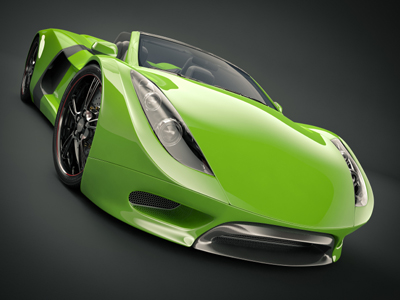What is the average speed of a car which takes 2 hours to travel 160 km?

# Speed 01

## A fascinating way to revise about calculating speed. Do you know how fast light travels?

### Scroll down to play the quiz

This is our first KS3 Science quiz regarding speed. The fastest possible speed at which energy or information can travel is the speed of light. Light travels incredibly fast. A ray of light can travel 300,000 km in one second! That means it can cover the distance from the Moon to Earth in 1 second and the 150 million km from the Sun to the Earth in just over 8 minutes.

It means that if the Sun suddenly stopped shining, we wouldn't know until a little over 8 minutes later. Despite this incredible speed, light from the most distant objects in the Universe has taken a mind-boggling 13.4 billion years to reach us. It is impossible to see the Universe as it is today, you always see it as it was in the past.

In order to calculate speed, you need to know the distance travelled and the time it takes.

The full scientific definition of speed is the distance travelled in unit time so it is therefore calculated by dividing distance by time taken to cover that distance. In SI units, the distance is measured in metres and the time in seconds. This means that the units of speed are metres per second which can be abbreviated to m/s. You will see speeds given in many other units too like kilometres per hour (which can be abbreviated to km/h or, if you are not writing in a scientific context - kph) and on British cars miles per hour (usually abbreviated to mph).

1.
The speed of a cyclist who rides 300 metres in 25 seconds is .......
0.12 m/s
12 m/s
24 m/s
7,500 m/s
300 m/25 s = 12 m/s
2.
The SI unit of speed is .......
cm/s
m/s
km/s
m/h
Speed is also measured in km/h, miles/h - you must make sure units are correct
3.
A bus takes 2 hours to travel 40,000 metres. The average speed of the bus in km/h is .......
2 km/h
20 km/h
33 km/h
200 km/h
40,000 m = 40 km so speed = 40/2 = 20 km/h
4.
An athlete runs 1 km in 100 seconds. Her speed in m/s is .......
0.001 m/s
1 m/s
10 m/s
100 m/s
1 km = 1,000 m so speed = 1,000 m/100 s = 10 m/s
5.
The speed of an athlete who runs 200 metres in 20 seconds is .......
0.1 m/s
10 m/s
10 km/s
4,000 m/s
200/20 = 10 m/s
6.
The average speed of a car which takes 2 hours to travel 160 km is .......
80 m/s
80 km/s
80 km/h
320 km/h
160 km/2 h = 80 km/h. Note how the units match those given in the question
7.
A child walks 90 metres in 3 minutes. The speed in m/s is .......
0.3 m/s
0.5 m/s
3 m/s
5 m/s
3 minutes = 180 seconds so speed = 90/180 = 0.5 m/s
8.
A car travels 20 km in 12 minutes. The speed in km/h is .......
0.2 km/h
100 km/h
120 km/h
240 km/h
12 minutes = 12/60 = 0.2 hours so 20 km/0.2 h = 100 km/h
9.
A cyclist travels 900 metres in 1 minute. The speed in m/s is .......
9 m/s
15 m/s
30 m/s
900 m/s
1 minute = 60 seconds so speed = 900/60 = 15 m/s
10.
It takes an hour to walk 1,800 metres up a steep hill. The speed in m/s is .......
0.5 m/s
1 m/s
1.8 m/s
2 m/s
1 hour = 60 minutes = 3,600 seconds so speed = 1,800/3,600 = 0.5 m/s